# VBA IF Statement

VBA in Excel stands for Visual Basic for Applications which is Microsoft’s programming language. To optimize the performance and reduce the time in Excel we need Macros and VBA is the tool used in the backend.

In this article, we are going to use how to use the If statement in Excel VBA.

### Implementation

In the Microsoft Excel tabs, select the Developer Tab. Initially, the Developer Tab may not be available.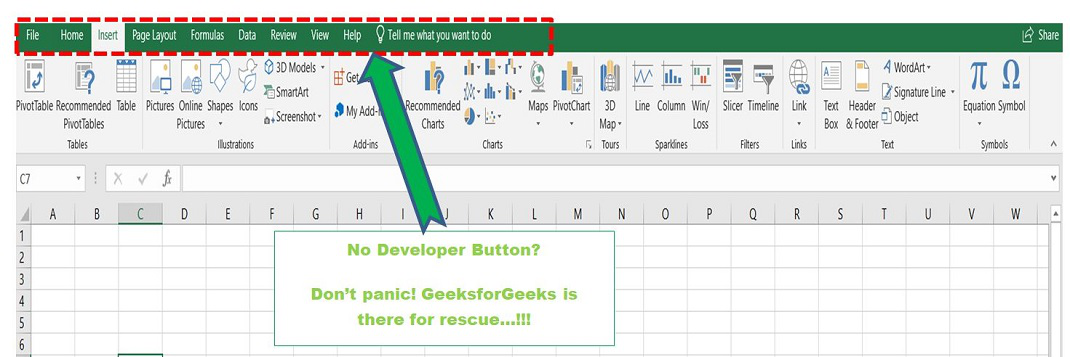The Developer Tab can be enabled easily by a two-step process :

• Right-click on any of the existing tabs at the top of the Excel window.
• Now select Customize the Ribbon from the pop-down menu.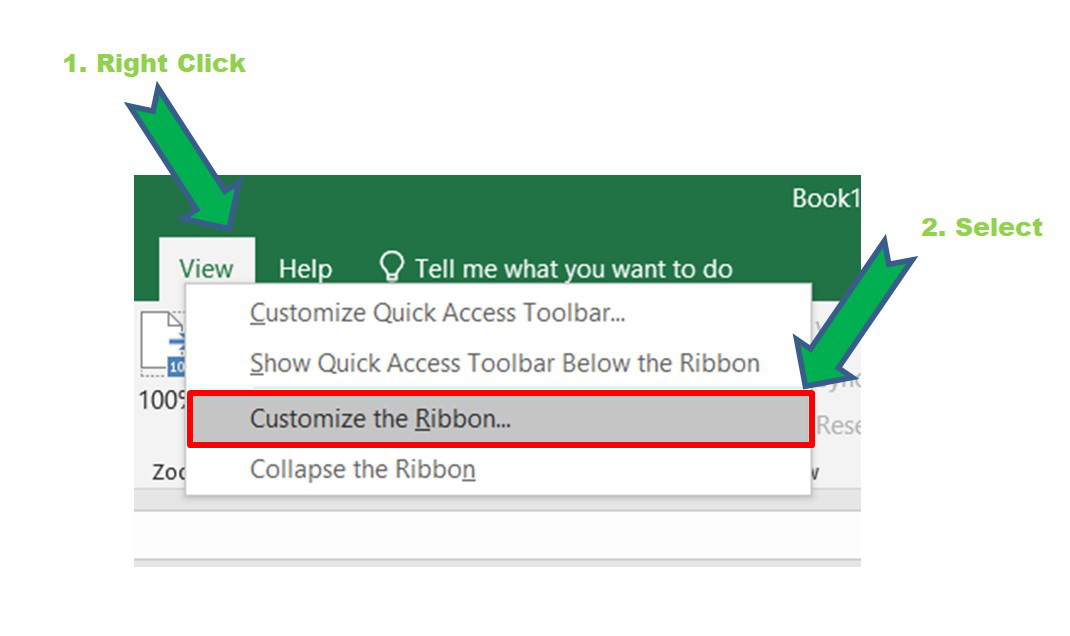• In the Excel Options Box, check the box Developer to enable it and click on OK.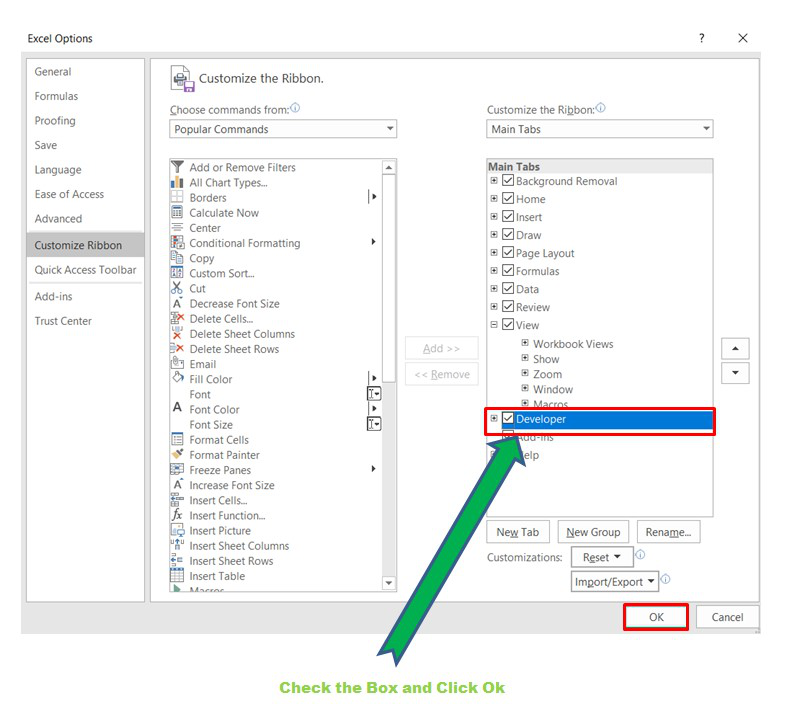• Now, the Developer Tab is visible.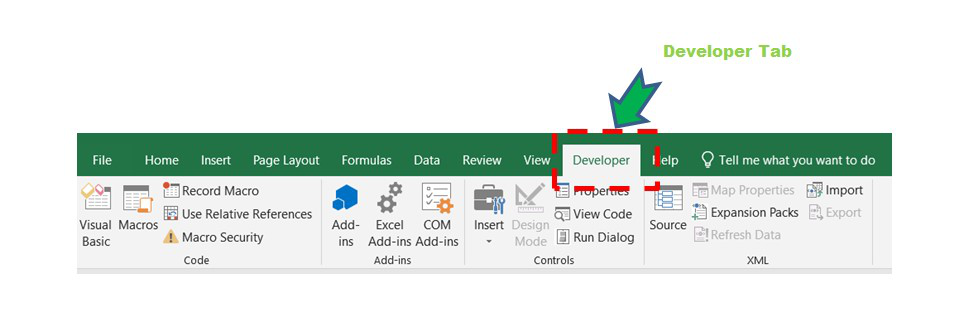Now click on the Visual Basic option in the Developer tab and make a new module to write the program using the Select Case statement.

Developer -> Visual Basic -> Tools -> Macros

• Now create a Macro and give any suitable name.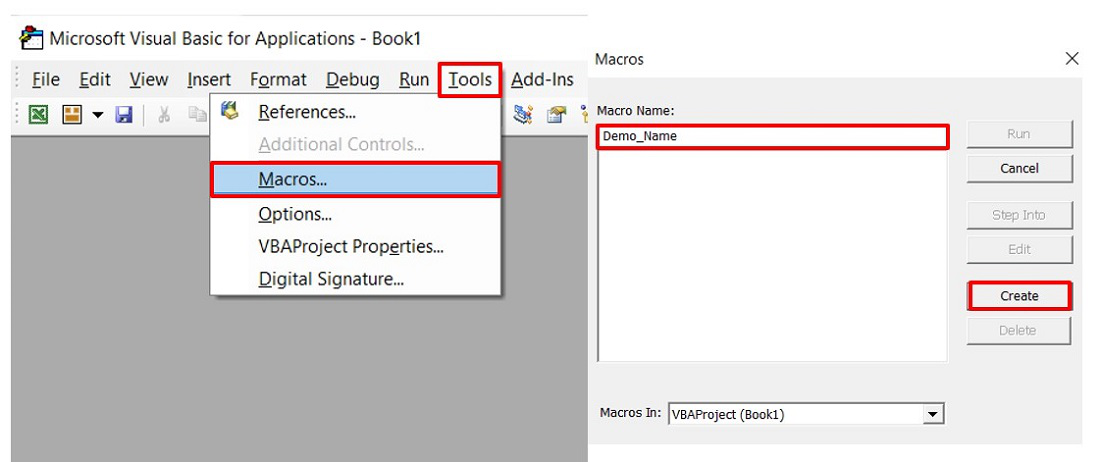• This will open the Editor window where can write the code.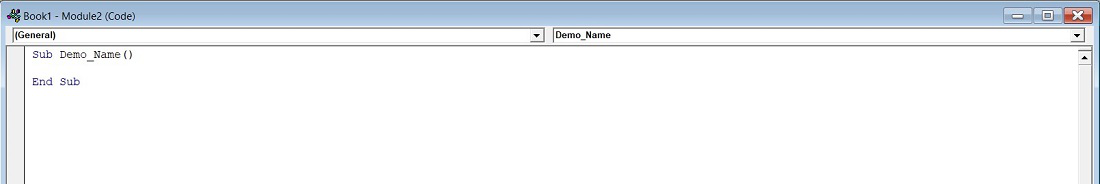## VBA IF Statement

The syntax is :

If condition/expression Then
Code Block for True value

Flow Diagram :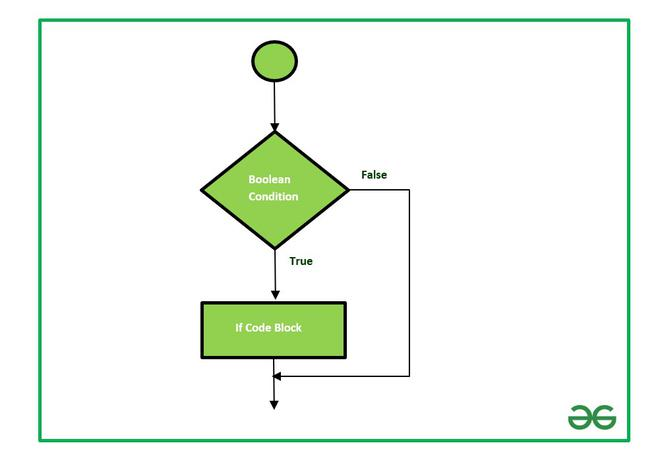Example: Consider a company that wants to hire employees for a certain role. The company kept eligibility criteria as the age of the person. The age of the candidate who can apply for this interview must be less than 27 years.

Sub Allocate_Employee()
‘Declaring and initializing the variable age
Dim age As Integer
age = 30
If age >= 27 Then
MsgBox “You are not eligible for this post.”
End If
End Sub

Output:

Since, the age is 30, the IF condition becomes TRUE and the code block inside IF statement executes.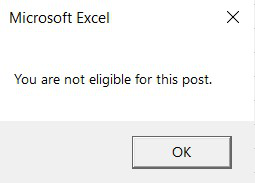Related Topic: Record Macros in Excel

## IF Then Statements in Excel

VBA If-Then Statements serve as conditional checks to determine whether expressions are TRUE or FAlse, enabling the execution of different sets of code based on the evaluation.

Let’s delve into a straightforward example to better understand this concept:

If Range(“A2”).Value >0 Then Range(“B2”).Value = “Positive”

In this case, the code tests whether the value within Range A2 is greater than zero. If this condition holds true, the code proceeds to set the value of Range B2 as “Positive”.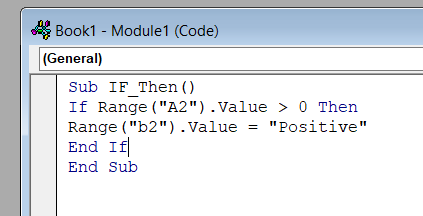Note: When assessing conditions, we employ comparison operators such as =,>,<,<>,<=, and >=. A more detailed exploration of these operators will follow later in this article.

Here’s the syntax for a concise single-line If statement:

If [test_expressions] Then [action]

To enhance readability, you can utilize a line continuation character(underscore) to spread the If statement across two lines, as demonstrated in the image above:

If [test_expression] then_

[action]

If Range(“a2”).Value >0 Then _

Range(“b2”).Value = “Positive”

## End If Statements

The previously illustrated “Single-line” If statement effectively addresses scenarios where you are testing a single condition. However, as your IF Statements grow more intricate, involving multiple conditions, it becomes imperative to incorporate an “End If” Statement to delineate the conclusion of the conditional logic.

For example, observe the following code:

If Range(“A2”).Value >0 Then

Range(“B2”).Value = “Positive”

End If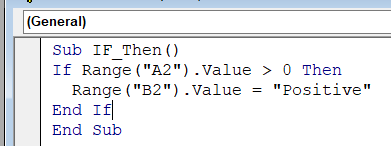Syntax:

If [test_expression] then

[action]

End If

Then End If signifies the end of the if statement.

## Using ElseIf with the VBA If Statement -Multiple Conditions

To address more nuanced scenarios involving multiple conditions, the ElseIf and Else Statements become integral components within your VBA code. The ElseIf statement supplements an existing If statement by assessing a condition only if the preceding conditions have not been met.

Consider the following illustration, where we expand upon the initial example of testing for a positive cell value by incorporating an ElseIf condition to determine i the value is negative:

If Range(“A2”).Value>0 Then

Range(“B2″).Value =”Positive”

ElseIf Range(“A2”).Value <0 Then

Range(“B2″).Value =” Negative”

End If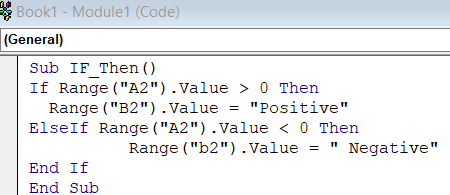Multiple ElseIf statements can be employed to accommodate varying conditions, enhancing the decision-making process. Take a look at this code snippet to comprehend its application:

Sub If_Multiple_Conditions()

If Range(“AA2″).Value =”Cat” Then

Range(“B2”).Value = “Kitten”

ElseIf Range (“A2”). Value = “Dog” Then

Range (“A2”).Value = “Puppy”

ElseIf Range (“A2”). Value = “Duck” Then

Range(“B2”).Value = “Duckling”

End If

End Sub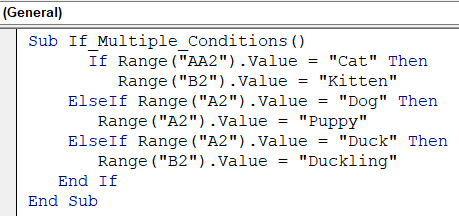By skillfully integrating ElseIf and Else statements, you adeptly navigate intricate decision paths within your VBA code, enabling you to respond dynamically to diverse scenarios and fostering heightened control and versatility in your Excel projects.

## Using Else With the VBA If Statement

For example, the Else statement steps in when neither the positive nor negative conditions hold true, indicating that the cell value must be zero. It serves as a vital catch-all mechanism, ensuring that a definitive outcome is assigned even when other specific conditions are unmet.

By incorporating the Else statement, you bestow your VBA code with the adaptability to handle a comprehensive spectrum of possibilities. Whether it’s dealing with unexpected inputs, exceptional scenarios, or default outcomes, the Else statement empowers your code to gracefully manage diverse circumstances and deliver meaningful results, enhancing the robustness and effectiveness of your Excel applications.

If Range(“a2”). Value>0 Then

Range(“b2”). Value = “Positive”

ElseIf Range(“a2”).Value< 0 Then

Range(“b2”).Value = “Negative”

Else

Range(“b2”).Value = “Zero”

End If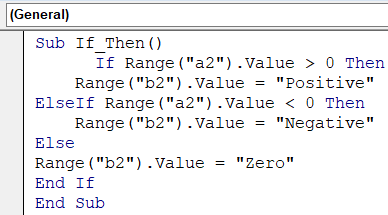## Using If-Else Statement in VBA

Among the fundamental constructs of VBA, the If-Else statement stands out as a versatile tool for decision-making within your Excel macros. This construct enables your code to assess conditions and respond dynamically, fostering tailored outcomes based on varying scenarios.

In a basic yet illustrative example, consider the following VBA code:

Sub If_Else()

If Range(“A2”).Value>0 Then

Range (“B2”).Value = “Positive”

Else

Range(“B2”).Value = “Not Positive”

End If

End Sub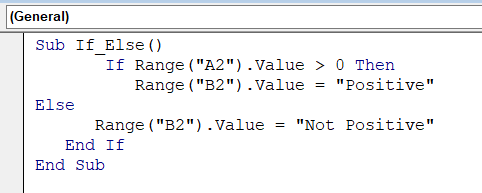In this code, the If-Else statements evaluate the value within Rabge A2. If the condition “Range(“A2″). Vaalue>0” holds true, it assigns “positive” to Range B2. Conversely, if the condition is false, it designates “Not Positive” to the same cell.

The If-Else statement embodies the essence of dynamic decision-making, enabling your VBA code to adapt and react based on real-time data. Its significance becomes pronounced as you tackle more intricate tasks, allowing you to handle diverse scenarios, and optimize the workflows, and functionality of your Excel spreadsheets.

By integrating If- Else statements into your VBA toolkit, you empower your macros to make informed choices and deliver relevant outcomes, thereby elevating the precision, efficiency, and intelligence of your Excel projects.

## Using Nested IFs Statements

Venturing beyond the confines of basic decision-making, VBA equips you with the capability to construct nested IF statements-empowering your code to explore multiple layers of conditions and responses.

The concept of nested IF statements is exemplified through the following VBA code:

Sub Nested_Ifs()

If Range(“A2”).Value >0 Then

Range(“B2″).Value =”Positive”

Else

If Range(“A2”).Value <0 Then

Range(“B2″).Value =”Negative”

Else

Range(“b2”).Value= “Zero”

End If

End If

End Sub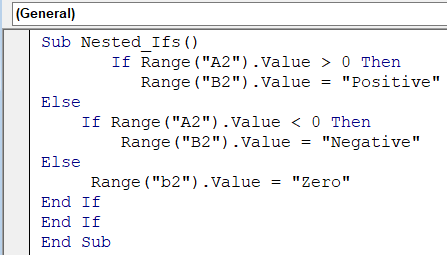In this example, a hierarchy of conditions is evaluated. Initially, the code assesses if the value within Range A2 is greater than Zero. Should this condition prove true,” Positive” is assigned to Range B2. In instances where the initial condition is False, the code then delves into a secondary nested IF statement. Here, it checks if the value is less than zero, and if so, assigns “Negative” to Range B2. Should both conditions remain unmet, the final ELSE statement designates “Zero” to the cell.

Nested IF statements grant you the power to navigate intricate decision trees, accommodating various scenarios and delivering precise outcomes. While the example demonstrates a binary hierarchy, you can further expand these constructs to accommodate multiple branches of logic, enhancing the adaptability and intelligence of your Excel macros.

By integrating nested IF statements into your VBA repertoire, you harness a sophisticated mechanism for managing complex conditions and refining the functionality of your Excel projects, contributing to enhanced decision-making and streamlined workflows.

## IF-Or, And, Xor, Not

In the realm of VBA, logical operators -Or, And, Xor, and Not -emerge as pivotal tools, fortifying your code with the ability to dissect complex conditions and execute dynamic decisions.

### If Or

The Or operator delves into the terrain of inclusivity, assessing whether at least one condition holds True. Observe how the following code scrutinizes a range’s value, gauging if it is less than 5,000 or surpasses 10,000:

If Range(“A2”).Value<5000 Or Range (“A2).Value> 10000 Then

Range(“B2”).Value= “Out of Range”

End If

If Range(“A2”). Value < 5000 or_

Range(“A2”). Value >10000 Or _

Range(“A2”).Value = 9999 Then

Range(“B2”).Value = “Out Of Range”

End If

### If And

In contrast, the And operator operates under the premise of totality, evaluating whether ALL conditions converge to true. Consider the ensuring illustration, where the code scrutinizes whether the range value resides within the range of 5,000 and 10,000:

If Range(“A2”). Value >=5000 And Range(“A2”). Value<=10000 Then

Range (“B2”). Value = “In Range”

End If

### If Xor

The Xor operator introduces a nuanced perspective, confirming if a singular condition exclusively holds true. Xor returns FALSE when zero conditions or multiple conditions are satisfied.

### If Not

If Not is used to convert the False to True or vice versa:

Sub If_Not()

MsgBox Not (True)

End Sub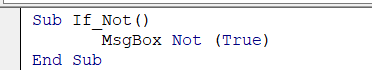## FAQs

### What is a VBA If Statements?

An If statement in VBA is a conditional statement that helps you to execute specific code based on whether a given condition is true or False. It forms the core of decision-making in VBA Programming.

### How do VBA If statements work?

VBA If statement evaluates a condition and, the code block will execute if the condition is true. And If the condition becomes False, the code block is skipped and the program continues with the next statement after the if block.

### What to do if none of the conditions are met in an If statement?

You can use the Else clause to specify a block of code to execute when none of the previous conditions are met.

Whether you're preparing for your first job interview or aiming to upskill in this ever-evolving tech landscape, GeeksforGeeks Courses are your key to success. We provide top-quality content at affordable prices, all geared towards accelerating your growth in a time-bound manner. Join the millions we've already empowered, and we're here to do the same for you. Don't miss out - check it out now!

Previous
Next